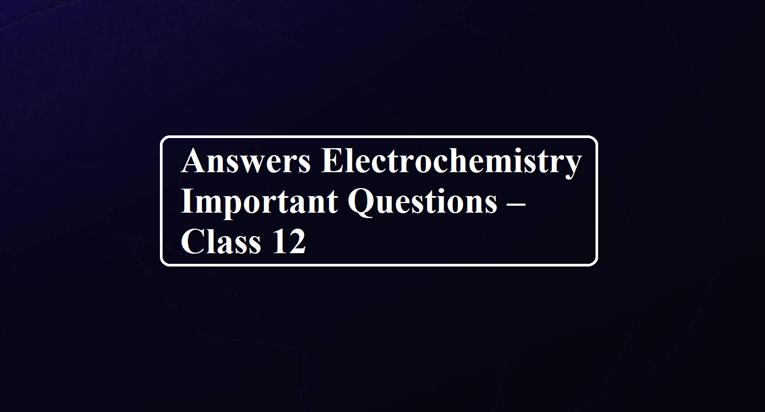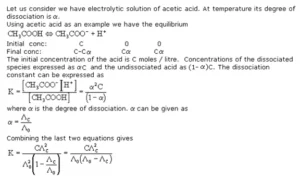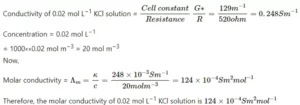# Answers Electrochemistry Important Questions – Class 12# Answers Electrochemistry Important Questions – Class 12

Ans 1. Cell stops working after some time because the electrode potential of both the electrodes becomes equal.

Ans 2. Ammonium Chloride (NH4Cl)

Ans 3. During the charging of lead storage batteries, it acts as an electrolytic cell and during the discharging of the lead storage battery, it acts as a galvanic cell. During the charging of the lead storage battery, the reactions get reversed and the cathode becomes the anode and the anode becomes the cathode.

Ans 4. A dry cell becomes dead after a long time even if it has not been used because the acidic NH4Cl corrodes the zinc container.

Ans 5. In a mercury cell, the anode is a zinc-mercury amalgam, the cathode is a paste of mercury (II) oxide and carbon, and the electrolyte is a moist paste of KOH-ZnO.
The cell reaction are as follows:
Anode: Zn(Hg) + 2OH → ZnO + H2O + 2e
Cathode: HgO + H2O + 2e → Hg + 2OH
Overall reaction: Zn + HgO → ZnO + Hg

Ans 6. A metal which is more electropositive than iron such as Al, Zn, and Mg can be used in cathodic protection of iron against rusting.

Ans 7. The reduction potential of aluminium is lower than that of water this means that it has a lesser tendency to get reduced than water.

Ans 8. Rusting of iron is due to the presence of H+ ions. An alkaline medium reduces the concentration of H+ ions by increasing the concentration of OH ions. It will neutralize H+ ions and inhibit rusting.

Ans 9.  On electrolysis
CaH2 → Ca2+ + 2H
At cathode (reduction):
Ca2+ + 2e → Ca
At anode (oxidation):
2H → H2 + 2e
At the anode, hydrogen is produced.

Ans 10.  Platinum adsorbs H2 where it remains in contact with H+ and thus, it provides a surface at which the exchange of electrons occurs.

Ans 11.  Strong electrolytes such as sodium chloride are present in saline water. The ions produced from NaCl help in the reduction of oxygen to form water. Hence, the rusting of iron is faster in saline water than in pure water.

Ans 12. Electrolysis: Electrolysis is the process that uses electric current to carry out a non-spontaneous chemical reaction.
In the electrolysis of molten NaCl, sodium ions migrate to the cathode.
Na+ + e → Na
Chloride ions migrate the other way, towards the anode.
Cl → 1/2 ​Cl2 ​+ e
The overall reaction is the breakdown of sodium chloride into its elements is:
2 NaCl → Na(s) + Cl2​(g)

Ans 13. The electrochemical equivalent of a substance is the mass of the substance deposited to one of the electrodes when a current of 1 ampere is passed for 1 second, i.e. a quantity of electricity of one coulomb is passed.
Overpotential: When a reaction is slow and has high activation energy then an extra potential is applied to carry out the non-spontaneous chemical reaction called over potential.

Ans 14. The advantages of H2 – O2 fuel cell over ordinary cells are:
(i) It has high efficiency and is eco-friendly.
(ii) The H2O produced can be used by astronauts for drinking purposes.

Ans 15. The conductivity of a solution is defined as the conductance of a solution of 1 cm in length and area of a cross-section of 1 sq. cm.
OR The inverse of resistivity is called conductivity or specific conductance.
OR The conductivity of a solution at any given concentration is the conductance (G) of one unit volume of solution kept between two platinum electrodes with the unit area of cross-section and at a distance of unit length.
Molar Conductivity: It is the conductance of a volume of solution containing 1 mole of dissolved electrolyte when placed between two parallel electrodes 1 cm apart.

Ans 16. In the corrosion reaction of iron, simultaneous oxidation and reduction occur in the object. Hence, it is an electrochemical reaction. The reactions occurring in rusting of iron are as follows.
Anode: 2 Fe(s) → 2Fe2+ + 4e
Cathode: O2 (g) + 4H+ (aq) + 4e- → 2 H2O(l)
Overall reaction: 2 Fe + O2 + 4 H+ → 2 Fe2+ + 2 H2O
Hydrate ferric oxide (Fe2O3. xH2O) in the form of rust is produced when ferrous ions produced are further oxidised by atmospheric oxygen. This is known as the rust.

Ans 17. Difference between metallic conductance and electrolytic conductance

 Metallic conductance Electrolytic conduction Conduction through metals is due to the movement of electrons. Conduction through electrolytes is due to the movement of ions. It does not involve any transfer of matter. It involves the transfer of matter as ions. No change in the chemical properties of the conductors is observed. It involves the chemical decomposition of the electrolytes. It decreases with the increase in temperature. It increases with the increase in temperature.

Ans 18. According to this law at infinite dilution when dissociation is complete each ion makes a definite contribution towards the value of limiting molar conductivity.Ans 19. We know
Ecell = E0cell – (0.0591/n) log [A0/A]
Given pH = 10
We know pH = – log [H+]
Conc. [H+] = 10-10 M
Ecell = E0cell – (0.0591/1) log [H2/H+]
Ecell = E0cell – (0.0591/1) log [1/10-10]
E0cell = 0
Ecell = – (0.0591/1) log [1/10-10]
Ecell = 0.591 V

Ans 20. Standard emf of a cell is the difference in the standard reduction potentials of cathode and anode when no current flows through the cell.

Ans 21. Advantages of fuel cell
(i) High efficiency
(iii) Pollution free

Ans 22.  Relation between cell constant G*, the resistance of the solution R and conductivity K of the solution is given by G* = RK
The relation between molar conductivity λm and conductivity k is given by
λm = k/C

Ans 23.  (i) Crude copper is made anode and a pure sheet of copper is made cathode,
The electrolyte is an aqueous solution of some copper(II) salt such as copper sulphate (CuSO4).
(ii) Anode loses weight due to oxidation of Cu and Fe atoms
Cu → Cu2+ + 2e
Fe → Fe2+ + 2e
Cathode gains weight due to deposition of Cu
Cu2+ + 2e → Cu
(iii) The loss and gain in the mass of electrodes are not equal because the anode loses mass due to the dissolution of copper ion (Cu2+), iron (Fe2+) and silver (Ag+) but the gain in mass is due to deposition of copper only. Hence gain in mass is slightly smaller as compared to the loss.
(iv) Silver will settle as anode mud, but Fe will remain in solution as Fe2+ ions.

Ans 24.  (i) The relation may be given as :
ΔG = − n F Ecell
If the redox reaction is under standard conditions, then
ΔG0 = − n F E0cell
Here nF is the quantity of charge passed. In case we want to obtain maximum work from the galvanic cell, then the charge has to be passed in a reversible manner. As we know the reversible work done by the galvanic cell is equal to the decrease in its Gibbs free energy.
(ii) Applications of electrochemical series
i) Oxidising and reducing strengths
ii) Comparison of reactivities of metals
iii) Calculation of emf of the cell
iv) Predicting feasibility of a redox reaction
v) Predicting the liberation of hydrogen gas from acids by metals.

Ans 25.  (a) Reasons:
(i) Strong electrolytes such as sodium chloride are present in saline water. The ions produced from NaCl help in the reduction of oxygen to form water. Hence, the rusting of iron is faster in saline water than in pure water.
(ii) Aluminium cannot be produced by the electrolysis of an aluminium salt dissolved in water because of the high reactivity of aluminium with the protons of water and the subsequent formation of hydrogen gas. As in an aqueous solution, protons (H+) are preferentially reduced before Al3 + ions, leading to hydrogen evolution.
(b) Given
Concentration of the KCl solution = 0.1 mol/L
Resistance of cell filled with 0.1 mole L-I  KCl solution =100 ohm
Cell Constant = G* = Conductivity × Resistance
= 1.29 × 10-2 ohm-1 cm-1 × 100 ohm = 1.29 cm-1 = 129 m-1
Cell constant for a particular conductivity cell